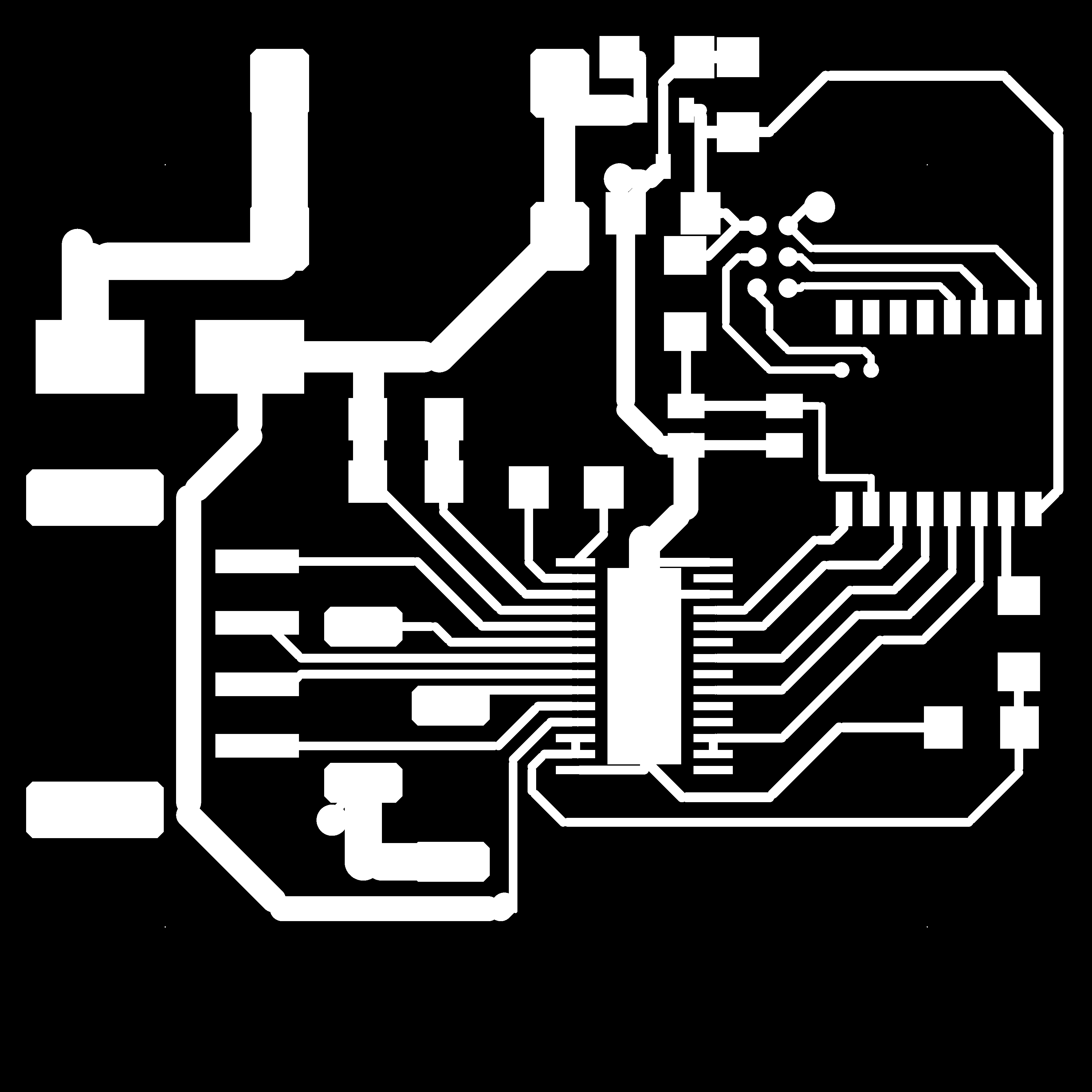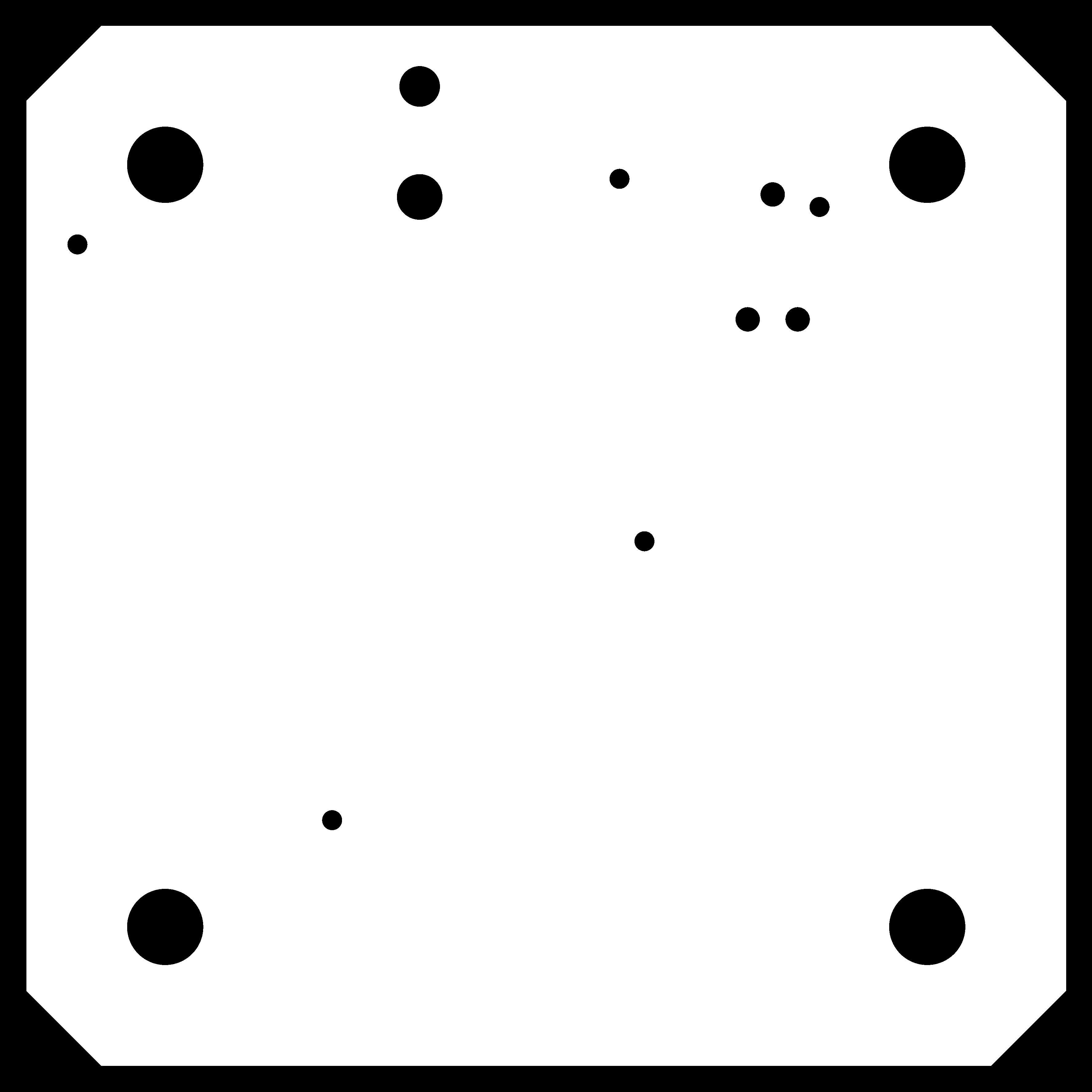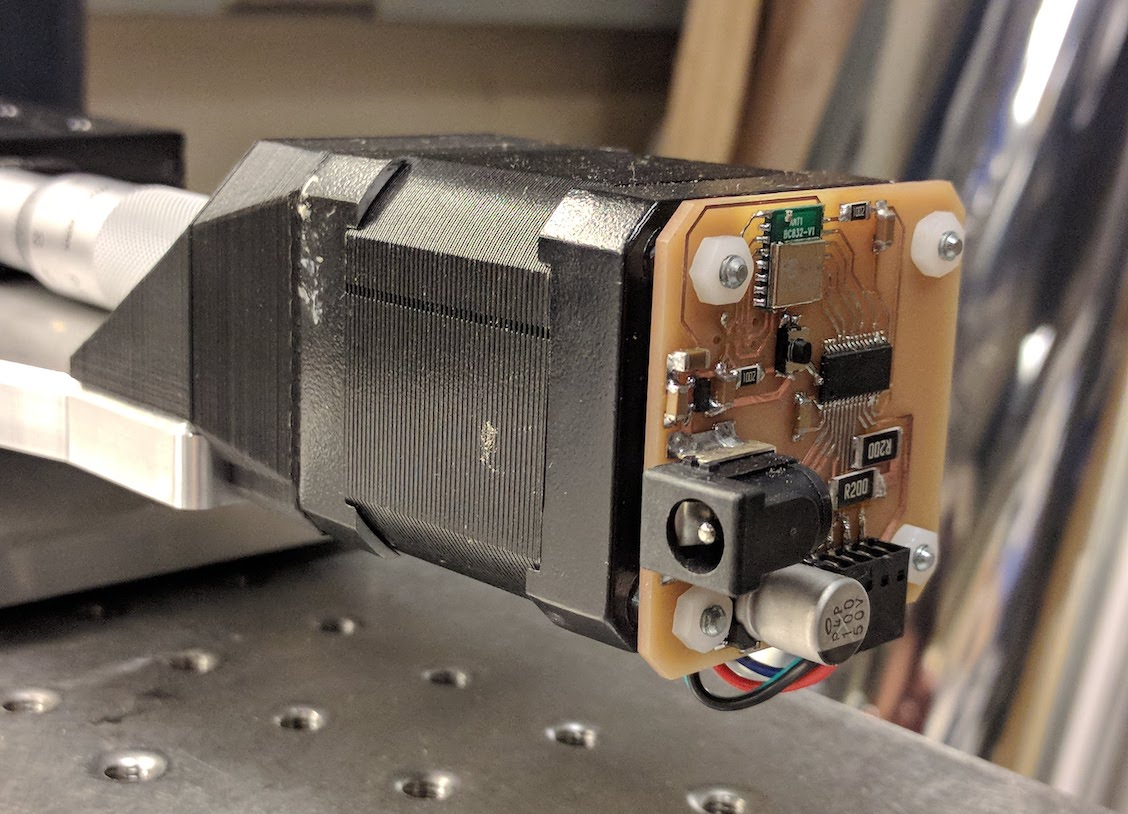# nRF52 + DRV8825 Stepper DriverThis is a stepper motor controller built around the DRV8825 and the nRF52. The nRF52 receives commands over the radio and controls the motor, which means we only need to deliver power to the motor, no data lines.

This board uses a PWM output and a low pass filter to deliver a voltage level to control the current limiting value for the DRV8825. A 10K resistor and a 1uF capacitor do a reasonably good job smoothing the 10kHz PWM into a constant voltage level.

Some sample firmware is available below (or as files: nrf52-drv8825.ino and radio.h). This code defines a command set issued over the radio for moving a number of steps, changing stepping speed, setting microstepping parameters, and setting a current limit value.

``````
uint16_t pwms = {0};
uint16_t step_period = 20000; //microseconds

const uint8_t pin_mode1 = 3; //A1
const uint8_t pin_mode0 = 0; //XL1
const uint8_t pin_step = 1; //XL2
const uint8_t pin_direction = 2; //A0
const uint8_t pin_nrst = 27; //p27
const uint8_t pin_ref = 26; //p26

int i=0; //counter

void stepper_setup(){
NRF_GPIO->DIRSET = (1 << pin_nrst); //set nrst/nslp pins as output
NRF_GPIO->OUTCLR = (1 << pin_nrst); //set nrst/nslp low to disable drv8825
NRF_GPIO->OUT = (0 << pin_nrst);

NRF_GPIO->DIRSET = (1 << pin_mode1) | (1 << pin_mode0); //set mode pins as output
NRF_GPIO->OUTCLR = (1 << pin_mode1) | (1 << pin_mode0); //set to full step mode

NRF_GPIO->DIRSET = (1 << pin_step) | (1 << pin_direction); //set step/dir pins as output

//Use PWM module to generate aref/bref
NRF_GPIO->DIRSET = (1 << pin_ref); //set ref pin as output
NRF_GPIO->OUTCLR = (1 << pin_ref); //set ref pin low
NRF_PWM0->PSEL.OUT = (pin_ref << PWM_PSEL_OUT_PIN_Pos) | (PWM_PSEL_OUT_CONNECT_Connected << PWM_PSEL_OUT_CONNECT_Pos); //set aref pin to pwm out
NRF_PWM0->ENABLE = (PWM_ENABLE_ENABLE_Enabled << PWM_ENABLE_ENABLE_Pos);
NRF_PWM0->MODE = (PWM_MODE_UPDOWN_Up << PWM_MODE_UPDOWN_Pos);
NRF_PWM0->PRESCALER = (PWM_PRESCALER_PRESCALER_DIV_1 << PWM_PRESCALER_PRESCALER_Pos); //16MHz tick
NRF_PWM0->COUNTERTOP = (1600 << PWM_COUNTERTOP_COUNTERTOP_Pos); //10 kHz pwm freq.
NRF_PWM0->LOOP = (PWM_LOOP_CNT_Disabled << PWM_LOOP_CNT_Pos);
pwms = 1600-100; //100/1600 * 3.3v = .2V = 200 mA limit
NRF_PWM0->SEQ.PTR = ((uint32_t)(pwms) << PWM_SEQ_PTR_PTR_Pos);
NRF_PWM0->SEQ.CNT = (1 << PWM_SEQ_CNT_CNT_Pos);
NRF_PWM0->SEQ.REFRESH = 0;
NRF_PWM0->SEQ.ENDDELAY = 0;
delay(1); //give aref filter time to settle.
}

void parse_command(){
//interpret command from radio for stepper actions
//move by commanded number of steps
NRF_GPIO->OUTSET = (1 << pin_direction); //set direction forwards
} else {
NRF_GPIO->OUTCLR = (1 << pin_direction); //set direction backwards
}
NRF_GPIO->OUTSET = (1 << pin_step);
delayMicroseconds(step_period);
NRF_GPIO->OUTCLR = (1 << pin_step);
delayMicroseconds(10);
}
} else if (radio_buffer == 2){
//change step speed
} else if (radio_buffer == 3){
//change microstepping
NRF_GPIO->OUTSET = (1 << pin_mode0);
} else {
NRF_GPIO->OUTCLR = (1 << pin_mode0);
}
NRF_GPIO->OUTSET = (1 << pin_mode1);
} else {
NRF_GPIO->OUTCLR = (1 << pin_mode1);
}
} else if (radio_buffer == 4){
//change current limit
pwms = 1600-radio_buffer; //100/1600 * 3.3v = .2V = 200 mA limit
NRF_PWM0->SEQ.REFRESH = 1;
}
else{
}

//unrecognized command, set radio buffer to all -1
//for(int i=0; i < PACKET_LENGTH; i++){
//}

for(i=0; i < PACKET_LENGTH; i++){
}
}

void setup() {

//Switch to internal LFCLK to disconnect from XL1 and XL2
NRF_CLOCK->LFCLKSRC = 0; //disconnect XL1 AND XL2 FROM LFCLK
NRF_CLOCK->EVENTS_LFCLKSTARTED = 0;
while (NRF_CLOCK->EVENTS_LFCLKSTARTED == 0) {}

stepper_setup();